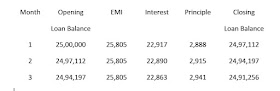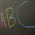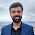## Saturday, 17 June 2017

### Break up of EMI into Interest and Principle | How is principal and interest calculated on EMI?

Loan borrowers are often confused why a major portion of EMI goes to interest and not the principal in the initial period of the loan

1. Is the Bank trying to make me pay more interest?
2. Is the Bank cheating me?
3. Why is such a big amount allocated to interest and not the principle?

These are the most common thoughts that come to a borrower who is a layman. His thoughts are justified as he doesn't know how the EMI gets divided between interest and principal

There can be 2 reasons for this-
(a) He has never asked the logic
(b) He has asked the rationale behind the bifurcation, but the banker has failed to explain the same to him

The good news is that there is no rocket science involved behind the division of EMI into principal and interest. It’s just a simple math.

Let us first understand what is EMI?

EMI stands for equated monthly installments. It is the amount that the borrower has to pay equally every month throughout the tenure of the loan such that the loan balance at the end of the tenure of the loan becomes zero. (Assuming that the borrower wouldn't make any prepayments during the course of the loan)

Why is there a need to calculate EMI?

Suppose you take a loan. Can you repay the loan as per your own whims and fancies?

The Answer is a big NO. Banks do not have a system to handle so many complex transactions where each borrower can repay the loan as per his/her convenience.

So it is important for banks to arrive at a figure which borrower can pay equally every month so that the loan gets repaid within the tenure of the loan.

So how is this EMI amount arrived at?

There is a slightly complex mathematical formula involved in the calculation of EMI amount.
You don’t need to manually calculate the EMI using the formula as there are lots of calculators available online where you just have to enter the value of the principal, the rate of interest per annum & tenure and you get EMI automatically. The formula is as given below

EMI = [P x R x (1+R)^N]/[(1+R)^N-1],

• P stands for the loan amount or principal,
• R is the interest rate per month. If the interest rate per annum is 11%, then the rate of interest per month will be 11% divided by 12 = (11/100)/12
• N is the number of monthly installments.

The above-mentioned formula gives you the amount which needs to be paid equally every month so that your loan gets over at the end of the tenure of the loan. (Assuming you wouldn't make any prepayments during the course of the loan)

For example, l
et's assume that-
The Loan amount is Rs.25, 00,000/-,
the Rate of interest is 11% and
Tenure of the loan is 20 years.

So,

P = Rs.25,00,000/-

R = Rate of interest per month
= (Annual Interest) divided by 12
= 11% divided by 12
= (11/100) divided by 12.

N = 240 months (In the formula N is number of months. So 20 years mean 240 months)

Applying the above formula, EMI will come to Rs.25, 805/-(Rounded off).

Now that we know the calculation of EMI, let’s understand the bifurcation of EMI between interest and principal

The formula of EMI calculates EMI in such a way that-
(a) At least the interest portion of every month gets covered fully in EMI &
(b) Principle amount gets divided over the period of the loan in such a way that the borrower has to pay a fixed amount (EMI) every month

The bifurcation of EMI into principle and interest is called amortization.

Now let’s go back to our same example. Let’s understand the bifurcation now

The bifurcation happens in the following manner-

1st Month
Loan Amount outstanding at the beginning of first month = Rs.25,00,000/-

Interest for the 1st month
= (11% of Rs.25,00,000) divided by 12
Rs.22,917/-.

(11% is the interest per annum. So to get the interest for one month, we need to divide the annual interest by 12)

The EMI arrived as per mathematical formula of EMI is Rs.25,805/- out of which Rs.22,917/- is the interest (Refer calculation of interest for 1st month above). The balance amount of Rs.2,888/- will go towards principal.

Balance amount

(EMI) minus (interest for the month)

= (Rs.25,805/-minus (Rs.22,917/-)

Rs.2,888/-

The principal portion in EMI is always equal to (EMI) minus (the interest for that particular month)

So the first month’s break up of EMI into interest and principal is as follows-

EMI = Rs.25,805/-

Interest = Rs.22,917/-

Principle = Rs.2,888/-(Balance amount remaining after deducting interest of the first month from EMI of the first month)

The loan amount outstanding at the end of the first month
= (Loan amount outstanding at the beginning of the first month) minus (principal repaid in first EMI)
= Rs.25,00,00/- minus (the principal portion of the first EMI)
= Rs.25,00,000/- minus Rs.2,888/-
= Rs.24,97,112/-Break up of Home Loan EMI into Interest and Principle

(Click and then enlarge the above image for better view)

2nd Month
The loan amount outstanding at the beginning of the second month

= The loan amount outstanding at the end of the first month
= Rs.24,97,112/-

In the second month, the interest will be calculated on Rs.24,97,112/- and not Rs.25,00,000/-. This is because the loan balance at the beginning of the second month is Rs.24, 97,112/-.

So interest for second month
= (11% of Rs.24,97,112/-) divided by 12
Rs.22,890/-.

The EMI of Rs,25,805/- will be the same for the entire tenure of the loan.

So balance amount of Rs.2,915/- will go towards the principal.

The Balance Amount of Rs.2,915/- is arrived as follows -
(
EMI)  minus (the interest for the second month)
= Rs.25,805 - Rs.22,890
= Rs.2,915/-

So the 2nd month’s EMI break up will be as follows-
EMI = Rs.25,805/-
Interest for 2nd Month = Rs.22,890/-
Principle (Balance Amount) = Rs.2,915/-

The loan amount outstanding at the end of 2nd month
= (Principal balance at the beginning of 2nd month) minus (principal portion of 2nd EMI)
= Rs.24,97,112/- minus (the principal portion of the second EMI)
=
Rs.24,97,112/- minus Rs.2,915/-
= Rs.24,94,197/-

3rd Month
The loan amount at the beginning of the third month
= The loan amount outstanding at the end of 2nd month
= Rs.24,94,197/- (as calculated above)

In the third month, the interest will be calculated on Rs.24,94,197/- (Outstanding Loan amount at the beginning of the 3rd month).

So interest for the 3rd month
= (11% of Rs.24,94,197/-) divided by 12
= Rs.22,863/-.

EMI for each month is Rs.25,805/-.

Hence principal portion of 3rd month's EMI
= Rs.25,805/- minus Rs.22,863/-
= Rs.2,941(Click and then enlarge the above image for better view)

If you continue bifurcating EMI for 20 years the way it is given above, you will find that each month, the interest portion of EMI keeps on decreasing and the principal portion keeps on increasing. This is because Loan amount outstanding is highest in the first month and then it keeps on reducing. Since the outstanding loan amount keeps on reducing after payment of each EMI, the interest portion of EMI also keeps decreasing. EMI will, however, remain the same for entire tenure and the amount of principal at the end of the 20th year will become zero.

This is how the EMI is bifurcated between Interest and Principal. Having gone through this, now you don't need anyone to explain to you why in the initial period, the major portion of EMI  goes towards interest, do you?

Related Posts -
Pre-Approved Home Loans
Factors that affect your home loan eligibility
Reasons behind rejection of a home loan

#### 8 comments:

1.Nice illustration. this blog made my ideas about home loan very clear. It will definitely help me to compare home loans of different banks.

1.2.Very well explained. Thank you.

1.3.Awesome explanation.After a tiring search I found tjis to be the best.

1.4.Hi,
It was really informative, thanks for sharing.
I have one doubt, if you could help me with that it would be really helpful.

I calculated emi, then bifurcated the principal and interest as mentioned above but on the 240th Month the value of outstanding balance goes in negative instead of zero.

Kindly check the calculations below and tell me if I'm wrong somewhere.

emi :25805
1 Interest : 22916.6667 Principal : 2888.3333 AMount: 2497112
2 Interest : 22890.1933 Principal : 2914.8067 AMount: 2494197
3 Interest : 22863.4725 Principal : 2941.5275 AMount: 2491255
.
.
.
238 Interest : 694.6408 Principal : 25110.3592 AMount: 50669
239 Interest : 464.4658 Principal : 25340.5342 AMount: 25328
240 Interest : 232.1733 Principal : 25572.8267 AMount: -245

1.Yes this happens due to rounding off of EMI amount. To avoid this, in last month don't wait for EMI to become due. In last month, go and pre-close the loan with whatever small amount is outstanding.